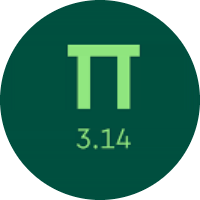# Learn the Place Value and Number Sense

In this quiz, you will find problems of converting numbers between standard form to expanded form, place values, comparing numbers, and roman numbers. You will also get to know about rounding off the numbers.

Start Quiz

What will be the standard form of the following equation?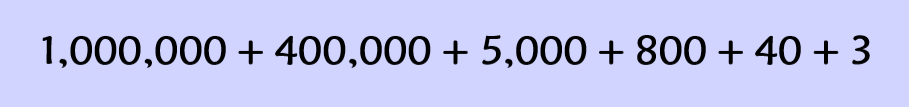104583

1405843

1450843

150483

Select the missing number.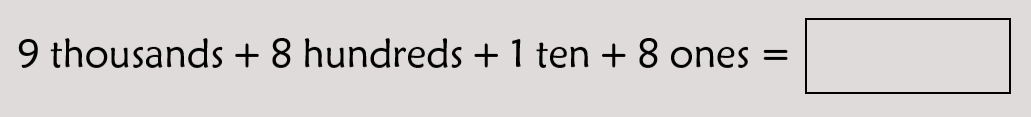9818

9188

9881

Which sign will make the following statement true?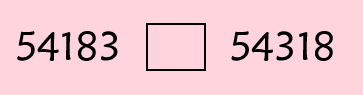>

<

=

How do you write this number using digits?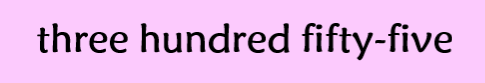355

553

175

375

How do you write this number using words?Eight thousand one hundred forty seven

Seven thousand four hundred seven

Eight thousand seven hundred forty one

Eight thousand four hundred forty

What number does this Roman numeral represent?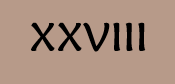27

17

18

28

What is \$911.65 rounded to the nearest 100 dollars?

\$900

\$1000

Is the subtraction of the following numbers even or odd?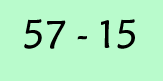Even

Odd

Select the standard form the following number.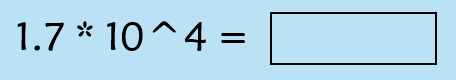170

1700

17000

Match the following.

Quiz/Test Summary
Title: Learn the Place Value and Number Sense
Questions: 10
Contributed by: